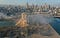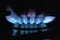# C programming is fun.

If you want to appreciate computer technology, you should try some simple computer programming. C is one of the most fundamental one.(Some of you may say Python).

It is still on the top list of TIOBE Index and in fact many computer scientists agree that C is their most favorite programming language.

During COVID-19, when you stay at home and do nothing, why not have a taste of computer language? You can install an IDE called Code::Blocks with GCC compiler here. (choose the MinGW version)

The most simple one is the “Hello World” program. …

# Ammonium nitrate and its storyBeirut, the largest city of Lebanon, was smashed seriously after the explosion of massive amount of ammonium nitrate.

Recently, there was a disaster happened in Beirut, Lebanon due to explosion of massive amount of ammonium nitrate that was stored in an inappropriate way. More than one hundred people died in the disaster. May his/her soul rest in peace.

In chemistry, preparation of ammonium nitrate involves neutralization of ammonia and nitric acid and meanwhile nitric acid is prepared by oxidation of ammonia. When you look into further, ammonia is synthesized by the well-known chemical process — Haber process. So, in total there are three stages.

In this medium article I would share with you the chemistry of ammonium nitrate.

# Solving Hess’ Law Problems

Hess’ Law states that the enthalpy change of a chemical reaction (physical process is also okay) is independent of the routes by which the reaction take place. But how does it work?Combustion of methane. Combustion is well-known an example of exothermic reaction. It gives a negative enthalpy change.

Origin of Hess’ Law

Well, the law is an expression of the simple but important law of conservation of energy — stating that energy is conserved; energy can neither be created nor destroyed, but only transfer from one form of energy to another form of energy. For example, combustion of methane involves energy transfer of chemical energy to heat.

Another reason is that “enthalpy” itself is a state…

# Degree of unsaturation/Double bond equivalence

This article shows a simple deviation of the double bond equivalence formula used in IB Chemistry or other equivalent chemistry syllabuses.

Double bond equivalence, or degree of unsaturation, is a formula used to determine the number of double bond, triple bond and ring structure present in the molecule of a given molecular formula. It is used in organic analysis to help us give a reasonable guess on the possible structure of an unknown organic molecule before using sophisticated instrumentation. …

# Balancing Redox Equation — Tips

This is my first article on chemistry. I would like to share the technique used in balancing redox equations.

There are two methods suggested in textbooks. They are

1. half equation(reaction) method
2. oxidation number method

Here, I illustrate the pros and cons of the method(s).

The advantage of the first method is that it does not require calculation of oxidation number and reduces the chance of mistake. For example, the oxidation number of Cr in dichromate ion, if you mis-calculate the result as +9, the whole redox equation will be wrong. However, you need to separate the unbalanced equation into oxidation…